## Introduction

Wild fluctuations in stock prices1,2,3,4,5,6,7,8 continue to have a huge impact on the world economy and the personal fortunes of millions, shedding light on the complex nature of financial and economic systems. For these systems, a truly gargantuan amount of pre-existing precise financial market data9,10,11 complemented by new big data ressources12,13,14,15 is available for analyses.

The complex mechanisms of financial market moves can lead to sudden trend switches16,17,18 in a number of stocks. Such sudden trend switches can occur in a synchronized fashion, in a large number of stocks simultaneously, or in an unsynchronized fashion, affecting only a few stocks at the same time.

Diversification in stock markets refers to the reduction of portfolio risk caused by the investment in a variety of stocks. If stock prices do not move up and down in perfect synchrony, a diversified portfolio will have less risk than the weighted average risk of its constituent stocks19,20. Hence it should be possible to reduce risk in price of individual stocks by the combination of an appropriate set of stocks. To identify such an appropriate set of stocks with anti-correlated price time series, the assumption mostly used is that the correlations among stocks are constant over time21,22,23,24,25,26. This widely used assumption is also the basis for the determination of capital requirements of financial institutions that usually own a huge variety of constituents belonging to different asset classes.

Recent studies building on the availability of huge and detailed data sets of financial markets have analyzed and modeled the static and dynamic behavior of this very complex system27,28,29,30,31,32,33,34,35,36,37,38,39, suggesting that financial markets are governed by systemic shifts and display non-equilibrium properties.

A very well known stylized fact of financial markets is the leverage effect, a term coined by Black to describe the negative correlation between past price returns and future realized volatilities in stock markets. According to Reigneron et al.40, the index leverage effect can be decomposed into a volatility effect and a correlation effect. In the course of recent financial market crises, this effect has regained center stage and the work of different groups has focused on uncovering its true nature40,41,42,43,44,45,46). Reigneron et al. analyzed daily returns of six indices from 2000 to 2010 and found that a downward index trends increase the average correlation between stocks, as quantified by measurements of eigenvalues of the conditional correlation matrix. They suggest that a quadratic term should be included to the linear regressions of the dependence of mean correlation on the index return the previous day.

Here, we will expand on these results utilizing 72 years of trading of the 30 Dow Jones industrial average (DJIA) components (see also47,48). Using this financial data set we will quantify state-dependent stock market correlations and analyze how they vary in face of dramatic market losses. In such “stress” scenarios, reliable correlations are most needed to protect the value of a portfolio against losses.

## Results

To quantify state-dependent correlations, we analyze historical daily closing prices of the N ≡ 30 components of the DJIA over 72 years, from 15 March 1939 until 31 December 2010, which can be downloaded as a Supplementary Dataset . During these T ≡ 18596 trading days, various adjustments of the DJIA occurred. We explicitly consider an adjustment of the index when one of the 30 stocks is removed from the index and replaced by a new stock in order to ensure that we accurately reproduce the index value of the DJIA at each trading day (Fig. 1).

To calculate the official index value pDJIA, the sum of prices of all 30 stocks is divided by a normalization factor dDJIA, known as the DJIA divisor. The DJIA divisor anticipates index jumps caused by effects of stock splits, bonus issues, dividends payouts or replacements of individual index components keeping the index value consistent (Fig. 1A). Consequently, the index value of the DJIA at day t is given by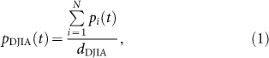where pi(t) reflects the price of DJIA component i at day t in units of USD and where t is measured in units of trading days. The normalization factor dDJIA is also measured in units of USD. Consequently, the value of the DJIA is dimensionless. Due to changes in the components of the DJIA, a component i does not necessarily reflect prices of one stock only. A subscript i is also used for a component's predecessor or successor.

To quantify state-dependent correlations, we calculate the mean value of Pearson product-moment correlation coefficients49 among all DJIA components in a time interval comprising Δt trading days each (Fig. 2). In each time interval comprising Δt trading day, we determine correlation coefficients for all pairs of N ≡ 30 stocks. From these correlation coefficients, we calculate their mean value for each time interval separately.

We relate mean correlation coefficients to corresponding market states, which we quantify by DJIA index returns for time intervals starting at trading day t and ending at trading day t + Δt,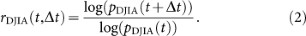We normalize the time series of DJIA index returns, rDJIA(t, Δt), by its standard deviation, σDJIAt), defined as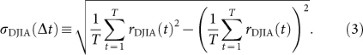The normalized time series of DJIA index returns, R(t, Δt), is given by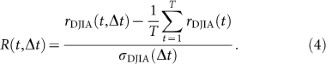In each time interval comprising Δt trading days, we calculate a local correlation matrix consisting of Pearson correlation coefficients49 capturing the dependencies among individual stock returns. Time-dependent returns of an individual stock i are given by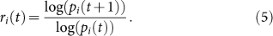In a Δt trading day interval, we calculate a correlation coefficient between return time series of stock i and return time series of stock j by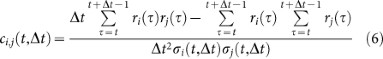with the standard deviation of return time series i determined in the same time interval comprising Δt trading days defined as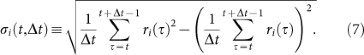The mean correlation coefficient of all DJIA components is given by the mean of all non-diagonal matrix elements of ci,j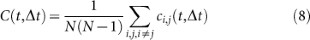Figure 3A depicts the relationship between normalized DJIA index return and corresponding mean correlation coefficient capturing the dependency amoung its components. Figure 3B depicts both normalized DJIA index returns and mean correlation coefficients which are used in our analysis for Δt = 10 days. Negative index returns tend to come with stronger correlation coefficients than positive index returns (Fig. 3A). Results for different time intervals Δt collapse into one single curve, suggesting a universal relationship.

To quantify the relationship between normalized index return and average correlation, we aggregate mean correlation coefficients for different values of Δt ranging from 10 trading days to 60 trading days (Fig. 4),We find consistency with two linear relationships quantifying the increase of the aggregated correlation C+ for positive index return R and the aggregated correlation C for negative index return R,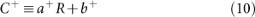with a+ = 0.064 ± 0.002 and b+ = 0.188 ± 0.004 (p-value < 0.001) quantifies the right part in Fig. 4A. The aggregatated correlations,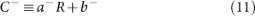with a = −0.085 ± 0.002 and b = 0.267 ± 0.005 (p–value < 0.001) quantifies the left part in Fig. 4A. The larger is a negative or positive DJIA return the larger is the corresponding mean correlation. In contrast, a reference scenario of randomly shuffled stock returns leads to a constant relationship (Fig. 4B), supporting our findings in Fig. 4A. However, this method destroys all correlations of this complex financial system and not only the link between aggregated correlation C* and normalized index returns R. As an additional test, we use non-shuffled time series of underlying stock returns for our analysis and randomly shuffle the DJIA return time series only (Fig. 4C). We find that the linear relationships reported in Fig. 4A also vanishes in this scenario highlighting the robustness of our findings.